Next: 6.2.3 RF Characteristics Up: 6.2 Power HEMT for 0.9/1.9 GHz and 40 GHz Applications Previous: 6.2.1 DC Characteristics

6.2.2 Dependence of Recess Geometry on the E-Field Distribution

The following investigations on breakdown will be carried out on the distribution of the electric field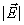in the channel for VDS = 10 V depending on VGS and geometry parameters. The electric field distribution reflects the resistance along a current path. The highest field is reached were the resistivity is high. The intrinsic transistor action is based on controlling the conductivity of the channel by the gate. As the series resitances are almost constant the relative resistivity along the current path is changed and thus the distribution offor a constant VDS.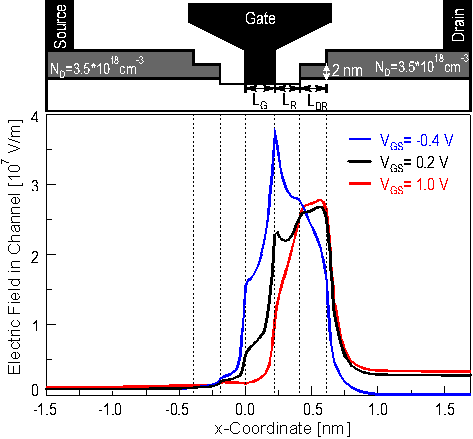Figure 6.24 Electric field distribution in the channel at VDS = 10.0 V.max is shifted from the drain end of the gate to the end of the double recess if the conductivity of the channel is increased by increasing VGS.

In  Figure 6.24 a cross section ofthrough the channel is shown for different bias points. For VGS < VT a peak is reached at the drain side of the gate because between gate and drain the largest voltage drop of VGD = 10.4 V occurs compared to VGS = ­0.4 V between gate and source. But the electric field is still quite high under the inner recess on the drain side which is characterized by LR. As the resistivity decreases significantly under the capis reduced accordingly. For higher VGS the conductivity in the channel is increased and the resistivity under the gate gets in the order of the resistivity under the inner and the double recess. Therefore the distribution ofis spread out accordingly and its maximum is decreased.max increases again in the open channel regime as the main voltage drop occurs only under the double recess area.

The comparison of the electric field distributions for different bias points leads to a design consideration of the recess geometry in respect to breakdown. The breakdown voltage for VGS < VT is mainly governed by the length LR of the inner recess whereas for open channel conditions the recessed cap becomes dominating. The recess in the cap is characterized by the thickness of the remaining highly doped cap layer and the double recess length LDR.

In  Figure 6.25 the electric field distribution for the open channel regime is shown for VGS = 0.8 V. If the remaining thickness dDR of the highly doped cap is zero basically the length of the inner recess is increased by LDR. In this casemax is reached at the end of the recess. If dDR is increased the resistive distribution is changed such thatis spread out more and thusmax is reduced. If dDR is to thickmax is still reached at the end of the inner recess and its magnitude is higher again.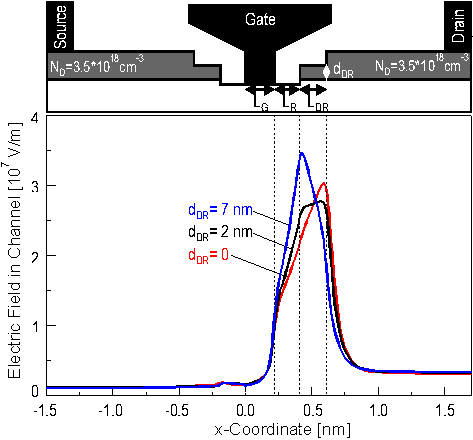Figure 6.25 Electric field distribution in the channel at VDS = 10 V and VGS = 0.8 V. To reducemax the thickness of the recessed cap has to be such thatis equally distributed under the recess.

It should be mentioned that the values for dDR given in  Figure 6.25 are unrealistically low. This is another indication that the model for the semiconductor/passivation interface might be not accurate enough. Additionally the depletion depth of the semiconductor surface highly depends on the damage as an inevitable consequence of the etching process.

To reducemax by increasing the series resistance in some areas leads directly to the trade-off between power capability and RF performance. In the following not only the impact of the geometry on the electric field distribution for VDS = 10 V will be investigated but also the consequences of the respective device geometry on gm and fT at VDS = 2.0 V and VGS = 0.4 (i. e. were gm max is reached).

In  Figure 6.26 the maximum field in the channel is shown versus the length of the inner recess LR for VDS = 10 V and VGS < VT. As depicted in the Figuremax decreases about linearly with LR. This goes along with an almost linear reduction of gm max for VDS = 2.0 V as the series resistance is increased.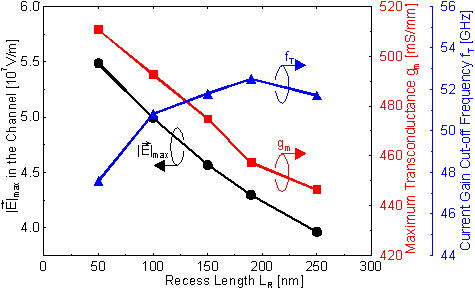Figure 6.26 The reduction ofmax at VDS = 10 V and VGS < VT is correlated with a reduction of gm at the active bias point VDS = 2.0 V and VGS = 0.4 V. A strong increase of CG leads to a reduced fT for small LR.

However, for the current gain cut-off frequency another effect becomes significant as already discussed for the low noise HEMTs in Section 6.1.2.2. With increasing LR the coupling between the contacts and thus CG is reduced. The reduction of CG is larger for small LR end gets less significant for larger LR. This overcompensates the linear reduction of gm for LR < 190 nm as shown in  Figure 6.26. The maximum fT of almost 53 GHz is reached for a significantly larger LR than in the case of the low noise HEMT. It will be discussed in Section 6.2.3.2 that the CG of the power HEMTs investigated here is very high such that the impact of a reduction of CG due to a larger LR is more significant than in the case of the low noise HEMTs.

When the channel starts to conduct current the double recess in the cap eventually becomes significant. It was shown in  Figure 6.25 that the thickness of the recessed cap dDR determines the location ofmax and thus influences its magnitude. In addition to the dependence ofmax on dDR the influence on the recess length LDR is shown in  Figure 6.27max can be controlled over a very wide range from above 4.5*107V/cm to below 2.0*107V/cm. For LR > 300 nmmax increases monotonously with the thickness dDR. For LR < 300 nm a local minimum appears. The minimum is shifted from dDR = 1 nm for LDR = 300 nm to dDR = 4 nm for LDR = 100 nm.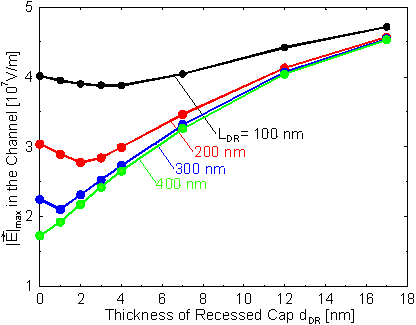Figure 6.27 Maximum electric field in the channel versus the thickness of the recessed cap dDR for an open channel bias point (VDS = 10 V, VGS = 0.8 V). Parameter is the length of that recess LDR.

The mechanism is illustrated in  Figure 6.25max without a double recess is about 5*107V/cm. Using a double recess with LR = 200 nm and dDR = 2 nmmax can be reduced by almost 50 % to 2.8*107V/cm. Figure 6.27 also indicates that to receive full benefit of the double recess a tight control of dDR is required. For too large dDRmax is reduced only slightly whereas for too small dDR not onlymax is increased again but additionally a larger series resistance has to be expected. A larger resistance manifests itself in a reduced gm as depicted in  Figure 6.28. If a double recess with LR = 200 nm and dDR = 8 nm is used instead of LR = 100 nm with the same dDRmax is already reduced substantially but the reduction in gm is still negligible. A double recess with LR = 400 nm and dDR = 2 nm reducesmax greatly but also gm is reduced by more than 10 %.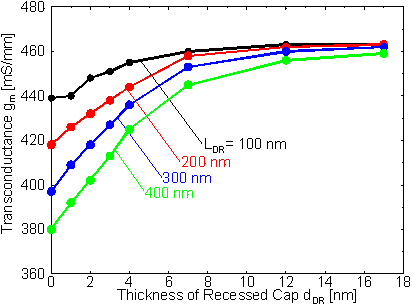Figure 6.28 Maximum transconductance gm versus the thickness of the recessed cap dDR at VDS = 2.0 V and VGS = 0.4 V. Parameter is the length of that recess LDR.

Similar to the length of the inner recess LR CG is influenced by the geometry of the recessed cap due to the coupling of the gate metal with the highly doped cap. The dependence of CG on dDR and LDR is shown in  Figure 6.29. CG is small if the recessed cap is fully depleted. But CG increases not only with the thickness dDR but also with decreasing LDR because in this case the coupling between the gate metal and the non recessed cap increases. The local maxima in the CG characteristics for LDR > 100 nm are not clear. It will be shown later in this chapter that the distance between the gate metal and the highly doped cap is quite small for this device, therefore, the coupling is very strong. In this case the uncertainties in the simulation due to effects such as quantization might become significant.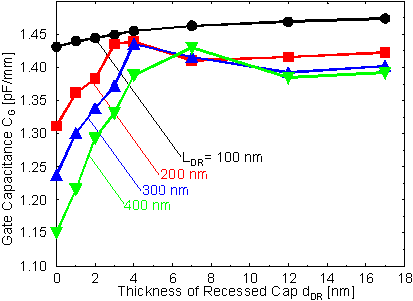Figure 6.29 Gate capacitance CG versus the thickness of the recessed cap dDR at VDS = 2.0 V and VGS = 0.4 V. Parameter is the length of that recess LDR.

This is getting even more pronounced in  Figure 6.30 where fT based on the data from  Figure 6.28 and  Figure 6.29 is shown. As both gm and CG are increased with increasing dDR and decreased with increasing LDR no large impact has to be expected on fT.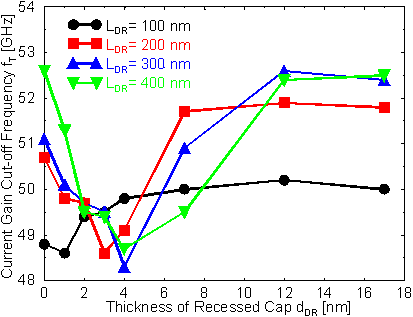Figure 6.30 Current gain cut-off frequency fT versus the thickness of the recessed cap dDR at VDS = 2.0 V and VGS = 0.4 V. Parameter is the length of that recess LDR.

For LDR = 100 nm again a weak local maximum can be observed similar to the dependence on the inner recess. The change of fT is only within 2 GHz. Some slightly larger effects have to be expected for LDR > 200 nm. The simulations in these cases are not accurate enough to clearly draw definite conclusions about the differences between LDR = 200 nm, 300 nm, and 400 nm. But common to all characteristics is that a local minimum appears for dDR between 3 nm and 4 nm. Even for variations of LDR and dDR in a very wide range fT is only changed within about 4 GHz.Next: 6.2.3 RF Characteristics Up: 6.2 Power HEMT for 0.9/1.9 GHz and 40 GHz Applications Previous: 6.2.1 DC Characteristics

Helmut Brech
1998-03-11